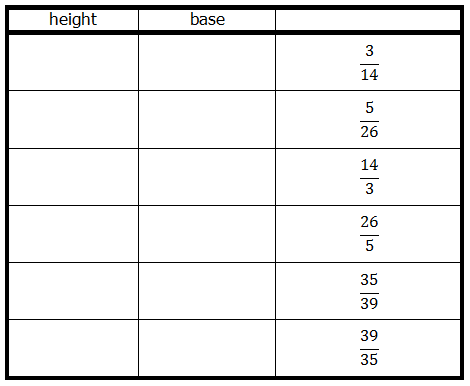# GMAT Two-Part Analysis

Two-part analysis

TPA(Two-Part Analysis) questions include a short paragraph with information. Multiple Answer choices are presented in different columns and rows where each column stands for a component and is a part of solution. You will have to select one answer from each column.

Example –

The area of a triangle, in square meters, is 7/3 times the height in meters, and it is also 13/5 times the base, in meters.In the table below, select a value for the height and value for the base, where both are measured in meters, so that the two values are jointly consistent with the information provided. Make only two selections, one in each column.

Join Free GMAT Webinar